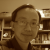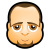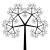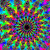Latest Blog Roll## Earth Cross Section

In the X3DOM mailing list, there was a discussion about constructing solid geometry. One application of solid geometry is to model the cross section of Earth. Unfortunately, solid geometry is not supported in X3DOM. However, the cross section can be achieved by using other geometry available in X3DOM. Here is an example.## Snub Dodecahedron

I have created a snub dodecahedron. The snub dodecahedron is an Archimedean solid.## Hexahedron Cube

I created a hexahedron (cube), which is a polyhedron platonic shape.## Archimedean solid- Truncated Cube

I have created the truncated cube which is an Archimedean solid. The truncated cube has 14 faces which are made up of 6 octagons and 8 triangles. The shape has 36 edges and 24 vertices.## Waves at sea

Following my previous blog about 3D function graphing, I did another experiment to animate the 3D graphs. In this blog, I used the same 3D function f(x,z) = x*z^3-z*x^3 to generate two sets of heights. Then a VECTORINTERPOLATOR (or VECINT) was used to animate this 3D graph. It looks to me like a wave on the sea surface. What do you think?